# How to Get the Position of the nth Occurrence of a Character in a Cell

In the previous post ,we talked that how to get the position of the last occurrence of a character in a cell, and sometimes, you may be want to know the position of the 2th, 3th or nth occurrence of a character in a text string in excel. and this post will guide you how to find the 2th, 3th, or nth occurrence of a character in a text string using excel formula and use defined function.

## Get the position of the nth occurrence of a character using excel formula

If you want to get the position of the nth occurrence of a character using a excel formula, you can use the FIND function in combination with the SUBSTITUTE function. For example, to get the position of the 2th occurrence of the character “e” in Cell B1, you can create the following formula:

`=FIND("#",SUBSTITUTE(B1,"e","#",2))`

The SUBSTITUTE function will replace the 2th occurrence of the character “e” with the hash character. So it returns another text string as “exc#l”.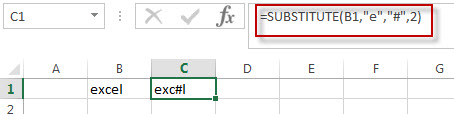The FIND function look up the hash character in the text string returned by the SUBSTITUTE function, and it returns the position of the first hash character in string “exc#l”. And it should be the position of the 2th occurrence of the character “e” in Cell B1.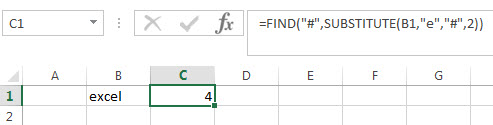## Get the position of the nth occurrence of a character using user defined Function

You can also create a new user defined function to get the position of the nth occurrence of a specific character or string in Excel VBA:

1# click on “Visual Basic” command under DEVELOPER Tab.2# then the “Visual Basic Editor” window will appear.

3# click “Insert” ->”Module” to create a new module named as: getNthPosition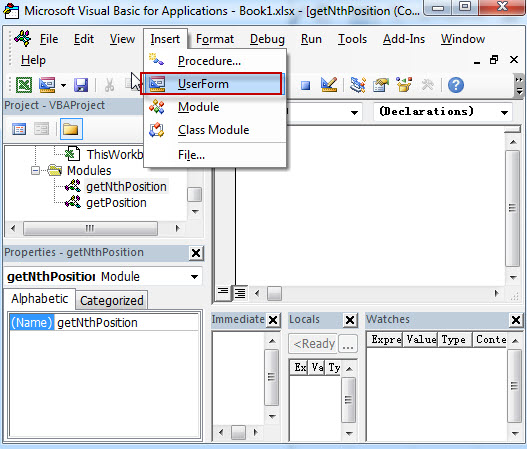4# paste the below VBA code into the code window. Then clicking “Save” button.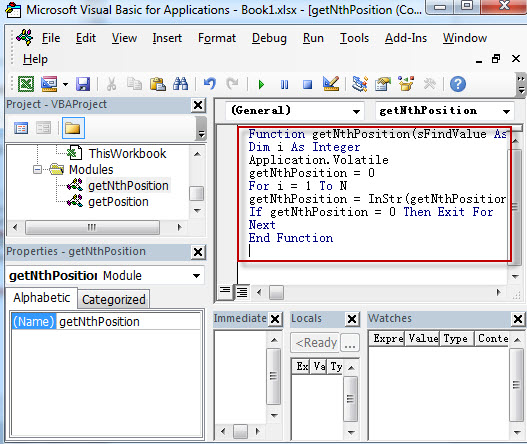```Function getNthPosition(sFindValue As String, sTextString As String, N As Integer) As Integer
Dim i As Integer
Application.Volatile
getNthPosition = 0
For i = 1 To N
getNthPosition = InStr(getNthPosition + 1, sTextString, sFindValue)
If getNthPosition = 0 Then Exit For
Next
End Function```

5# back to the current workbook, then enter the below formula in Cell C1:

`=getNthPosition("e",B1,2)`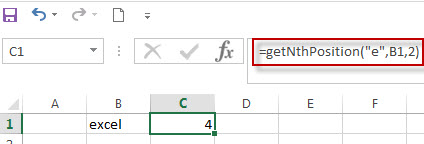### Related Formulas

• Get the position of Last Occurrence of a value in a column
If you want to find the position number of the last occurrence of a specific value in a column (a single range), you can use an array formula with a combination of the MAX function, IF function, ROW function and INDEX Function.
•  Get the position of Last Occurrence of a character or string in a cell
If you want to get the position of the last occurrence of a character in a cell, then you can use a combination of the LOOKUP function, the MID function, the ROW function, the INDIRECT function and the LEN function to create an excel formula.…
• Combine Text from Two or More Cells into One Cell
If you want to combine text from multiple cells into one cell and you can use the Ampersand (&) symbol.If you are using the excel 2016, then you can use a new function TEXTJOIN function to combine text from multiple cells…
• Split Text String to an Array
If you want to convert a text string into an array that split each character in text as an element, you can use an excel formula to achieve this result. the below will guide you how to use a combination of the MID function, the ROW function, the INDIRECT function and the LEN function to split a string…
• Find the Relative Position in a Range or Table
If you want to know the relative row position for all rows in an Excel Range (B3:D6), you can use a excel Array formula as follows:=ROW(B3:D6)- ROW(B3) + 1. You can also use another excel array formula to get the same result as follows:=ROW(B3:D6)-ROW(INDEX(B3:D6,1,1))+1…
• Get the First Row Number in a Range
If the ROW function use a Range as its argument, it only returns the first row number.You can also use the ROW function within the MIN function to get the first row number in a range. You can also use the INDEX function to get the reference of the first row in a range, then combined to the ROW function to get the first row number of a range.…
•  Get the Last Row Number in a Range
If you want to get the last row number in a range, you need to know the first row number and the total rows number of a range, then perform the addition operation, then subtract 1, the last result is the last row number for that range.…

### Related Functions

• Excel ROW function
The Excel ROW function returns the row number of a cell reference.The ROW function is a build-in function in Microsoft Excel and it is categorized as a Lookup and Reference Function.The syntax of the ROW function is as below:= ROW ([reference])….
• Excel Substitute function
The Excel SUBSTITUTE function replaces a new text string for an old text string in a text string. The syntax of the SUBSTITUTE function is as below:= SUBSTITUTE  (text, old_text, new_text,[instance_num])….
• Excel Find function
The Excel FIND function returns the position of the first text string (substring) from the first character of the second text string. The FIND function is a build-in function in Microsoft Excel and it is categorized as a Text Function. = FIND(find_text, within_text,[start_num])…
Related Posts

How to Extract First Letter from Each Word in a Cell in Excel

This post will guide you how to extract first letter from each word in a given cell in Excel. How do I extract the first letter of each word in a range of names in your current worksheet in Microsoft ...

How to ignore Blank Cells while Concatenating cells in Excel

This post will guide you how to concatenate cells but ignore all blank cells in your worksheet in Excel. How do I concatenate cells but ignore blank cells with a formula in Excel. How to create a concatenate formula to ...

How to Calculate the Average Excluding the Smallest & Highest Numbers in Excel

Calculating the average for a batch of data is frequently used in our daily life. But for some cases like statistic the average score in a competition, or price analysis, we often calculate the average excluding the smallest and highest ...

How to Get the Maximum or Minimum Absolute Value in Excel

It is easy to find the maximum or minimum value in a batch of data in excel, but if this batch of numbers contains both positive and negative numbers, the maximum or minimum absolute value cannot be found out by ...

How to Count Only Numbers in Bold in a Range of Cells in Excel

This post will guide you how to Count only numbers with bold style in a range of cells in Excel. How do I Count on cells with bold font within a range of cells using User defined function in Excel ...